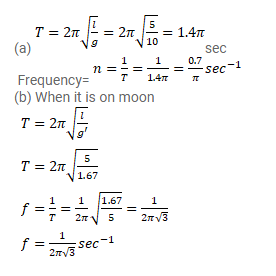# A simple pendulum is constructed by hanging a heavy ball by

Question:

A simple pendulum is constructed by hanging a heavy ball by a $5.0 \mathrm{~m}$ long string. It undergoes small oscillations.

(a) How many oscillations does it make per second?

(b) What will be the frequency if the system is taken on the moon where acceleration due to gravitation of the moon $1.67 \mathrm{~m} / \mathrm{s}^{2}$.

Solution: